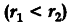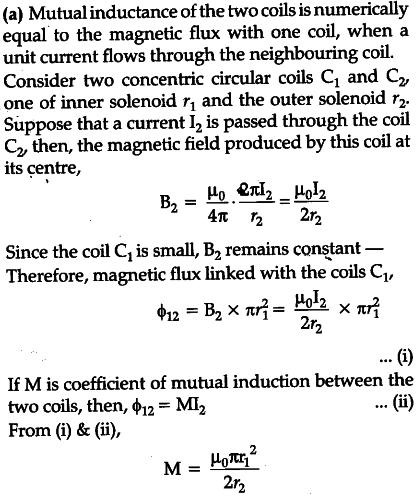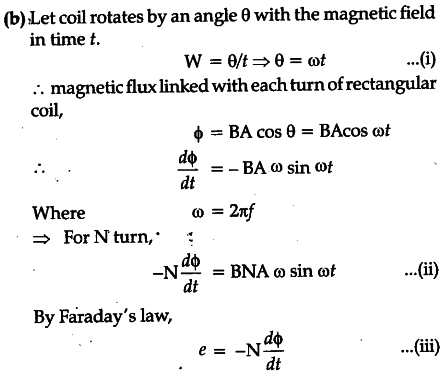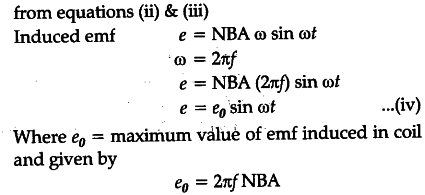# Explain the meaning of the term mutual inductance

(a) Explain the meaning of the term mutual inductance. Consider two concentric circular coils, one of radius {{r}_{1}}
and the other of radius {{r}_{2}}placed co-axially with centres coinciding with each other. Obtain the expression for the mutual inductance of the arrangement.
(b) A rectangular coil of area A, having number of turns N is rotated at 'f revolutions per second in a uniform magnetic field B, the field being perpendicular to the coil. Prove that the maximum emf induced in the coil is 2\pi f NBA.